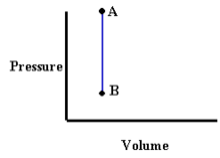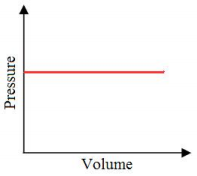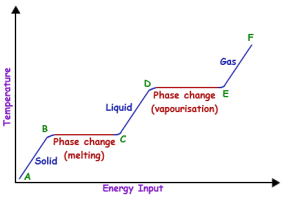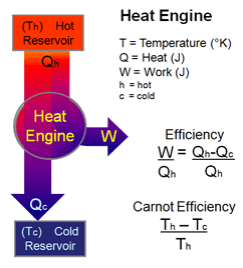×

×

CLEMSON / Physics / PHYS 2080 / How to find the temperature in fahrenheit?

# How to find the temperature in fahrenheit? Description

##### Description: This study guide includes important equations, main topics, important values and charts, and suggested practice problems from the textbook. This material comes from Chapters 16, 17, and 18.
8 Pages 174 Views 3 Unlocks
Reviews

Physics 2080

## How to find the temperature in fahrenheit?Exam 1 Study Guide

Chapters 16-18

Exam Date: 1-28-2016

Amanda Biddlecome

Equations

1) How to find temperature in Fahrenheit:

TF=(9/5)Tc+32°

*TF=Fahrenheit Temperature *Tc=Celsius Temperature

2) How to find temperature in Celsius:

TC=(TF-32)(5/9)

*TC=Celsius Temperature *TF=Fahrenheit Temperature

3) How to find temperature in Kelvin:

TK=TC+273.15

## How to find the temperature in celsius?*TK=Kelvin Temperature *TC=Celsius Temperature

4) Thermal Expansion (Linear)

*ΔT is the same for Kelvin and Celsius

*α can be represented as either Kelvin or Celsius and varies by material  ΔL=αLΔT

*ΔL=change in length

*α=coefficients of linear expansion (Celsius degrees)-1 *L=length  *ΔT=change in temperature If you want to learn more check out Expensive income statements refer to what?

5) Thermal Expansion (Volume)

*ΔT is the same for Kelvin and Celsius

*β varies by material

ΔV=βVΔT

*ΔV=change in volume         *β=coefficient of volume expansion=3α (Celsius)-1 *V=volume  *ΔT=change in temperature

## How to find the temperature in kelvin?6) Rate of conduction of heat across a temperature difference

*ΔT is the same in Kelvin and Celsius

*k varies by material

Q/Δt=(kA/L)ΔT

*Q=heat: J(smC)-1    *Δt=change in time (s)

*k=thermal conductivity of the material (W/(m-K)) *A=cross-sectional area (m) *ΔT=change in temperature (K)We also discuss several other topics like What is the definition of rhetoric?

7) The amount of energy radiated by an object

*emissivity is a number between 0-1 and is unit-less We also discuss several other topics like What is staphylococcus aureus?

P=eσT4A

*P=energy radiated (W) *e=emissivity  Don't forget about the age old question of What is the meaning of the string revolution?
Don't forget about the age old question of What are the different forms of a business organization?

*σ=Stefan-Boltzmann constant=5.67X10-8 Wm-2K-4  *T=temperature (K) *A=surface area (m)

8) Ideal Gas Law when given the number of moles

*Be careful with the units in these values, especially pressure

PV=nRT

*P=pressure (atm or kPa or Pa) *V=volume *n=number of moles  *R=universal gas constant=8.31 J(molK)-1  *T=temperature (K)

9) Ideal Gas Law when given the number of molecules

*Be careful of the units in these values, especially pressure

PV=NkBT

*P=pressure (atm or kPa or Pa)  *V=volume  *N=number of molecules  *kB=Boltzmann’s constant=1.38X10-23JK-1  *T=temperature (K) Don't forget about the age old question of Noncovalent bonding refers to what?

10) rms speed

vrms=√[(v12+v22+…+vn2)/n]

*vrms=rms speed  *n=number of moles

11) Average velocity

v=(v1+v2+…+vn)/n

*v=average speed  *n=number of moles

12) Average Kinetic Energy

Kavg=(1/2)mv2rms

*Kavg=averagen kinetic energy  *m=mass  *vrms=rms speed

13) Average Kinetic Energy

Kavg=(3/2)kT

*Kavg=average kinetic energy  *k=Boltzman’s constant=1.38X10-23J/K  *T=temperature (K)

14) rms speed

*this is a combination of the two Average Kinetic Energy equations

vrms=√[(3kT)/m]

*vrms=rms speed  *k=Boltzman’s constant=1.38X10-23J/K  *T=temperature (K) *m=mass

15) Internal Energy

U=(3/2)NkT=(3/2)nRT

*U=internal energy (J)  *N=number of molecules

*k=Boltzman’s constant=1.38X10-23J/K  *T=temperature (K)

*n=number of moles *R=universal gas constant=8.31J/(molK)

16) The amount of heat required to raise a mass’s temperature

*Be sure the units in the specific heat match the units of mass

*ΔT is the same in Kelvin and Celsius

Q=mcΔT

*Q=heat  *m=mass  *c=specific heat c(J/kgK)  *ΔT=change in temperature

17) The amount of heat required to change a mass’s phase

Q=mL

*Q=heat  *m=mass

*L=latent heat (fusion, vaporization, or sublimination) (Jkg-1)

18) Change in internal energy

ΔU=Uf-Ui

*ΔU=change in internal energy (J) *Uf=final internal energy (J)

*Ui=initial internal energy (J)

19) Change in internal energy

*The signs in this problem are very important and can be tricky, so read the problem well ΔU=Q-W

ΔU=change in internal energy (J)  *Q=heat (J)  *W=work (J)

20) Work done by an expanding gas at constant pressure

*When you have a constant volume, the work will be 0

W=PΔV

*W=work (J)  *P=pressure  *V=volume

21) Work found by interpreting a PV Diagram

W=NkTln(Vf/Vi)=nRTln(Vf/Vi)

*W=work  *N=number of molecules  *k=Boltzman’s Constant

*T=temperature  *ln=natural log  *Vf=final volume  *Vi=initial volume  *n=number of moles   *R=universal gas constant

22) Heat released at a constant volume

Qv=nCvΔT

*Qv=heat at a constant volume  *n=number of moles

*Cv=specific heat at a constant volume  *ΔT=change in temperature

23) Specific Heat at a constant volume

Cv=(3/2)R

*Cv=specific heat at a constant volume  *R=universal gas constant

24) Heat released at a constant pressure

Qp=nCpΔT

*Qp=heat at a constant pressure *n=number of moles

*Cp=specific heat at a constant pressure  *ΔT=change in temperature

25) Specific Heat at a constant pressure

Cp=(5/2)R

*Cp=specific heat at a constant pressure  *R=universal gas constant

26) Work done by a heat engine

W=Qh-Qc

*W=work  *Qh=heat released at hot temperature

*Qc=heat released at low temperature

27) Efficiency of a heat engine

e=1-(Qc/Qh)

*e=efficiency  *Qc=heat released at cold temperature

*Qh=heat released at hot temperature

28) Heat and temperature relation

*the temperatures Tc and Th are ALWAYS in Kelvin

(Qc/Qh)=(Tc/Th)

*Qc=heat released at cold temperature

*Qh=heat released at hot temperature  *Tc=cold temperature

*Th=hot temperature

29) Efficiency of a Carnot engine

ecarnot=1-(Tc/Th)

*ecarnot=efficiency of a Carnot engine  *Tc=cold temperature

*Th=hot temperature

30) Maximum work a heat engine can do

Wmax=[1-(Tc/Th)Qh]

*Wmax=maximum work  *Tc=cold temperature  *Th=hot temperature  *Qh=heat released at hot temperature

Main Ideas

-Thermodynamics

-Temperature

*heat, thermal contact, thermal equilibrium, Zeroth Law of Thermodynamics,  Fahrenheit to Celsius to Kelvin conversions, how pressure and temperature  are  related, absolute zero

-Thermal Expansion

*calculations, Volume Thermal Expansion and Linear Thermal Expansion -Heat

*calories, spontaneous heat flow, units, internal energy, conduction,  convection,  radiation

-Thermodynamic Processes

*ideal gas, pv diagrams, isovolumic process, isobaric process, Boyle’s Law,  adiabatic process

-Ideal Gas Law

*different equations for moles and molecules

-Units of measurement

-Kinetic Theory

*what it relates, how molecules act in a container, pressure, different types of  speed and how to calculate them, kinetic energy, potential energy, internal  energy  -Heat

*specific heats, latent heat, phase change diagrams, how to find total heat,  difference in heat used to change the temperature and heat used to change  the  phase

-Zeroth Law of Thermodynamics

*what it is

-First Law of Thermodynamics

*equations, constant volume, how internal energy and temperature relate,  quasi static systems, reversible systems, idealized reversible processes,  constant pressure  and changing volume in relation to work, free expansion,  adiabatic processes and  how they relate to the first law

-Specific Heats

*how to find them at constant volume and constant pressure

-Second Law of Thermodynamics

*spontaneous heat flow, heat engines, work related to heat engines,  efficiency  related to heat engines, temperature related to efficiency and work,  heat engines that  work backwards, heat pumps

-Third Law of Thermodynamics

*Absolute Zero

Common Diagrams and Values

-Absolute Zero=0 Kelvin

-Freezing point of water=32°F, 0°C, 273 K

-Boiling point of water=212°F, 100°C, 373 K

-Specific Heat of water (c)=4.186 J/gram°C

-Stefan-Boltzmann constant (σ)=5.67X10-8Wm-2K-4

-Universal Gas Constant (R)=8.31 J(molK)-1

-Botzmann’s Constant (kb)=1.38X10-23JK-1

-Room Temperature=20°C, 68°F, 283 K

-Gamma (γ)=5/3

-Specific Heat at Constant Pressure (Cp)=(5/2)R

-Specific Heat at Constant Volume (Cv)=(3/2)R -Isovolumetric Process-Isobaric Process-Isothermal Process (Boyle’s Law) and Adiabatic Process-Phase Change Diagram-Heat Engine DiagramChapter 16:

-PG 565, Conceptual Questions:

*1, 2, 8, 10, 11, 12, 15, 16

-PG 566-569, Problems and Conceptual Exercises:

*1-8, 12-14, 17-22, 24, 25, 33-39, 41-43, 46, 49-52, 54-56, 59

Chapter 17:

-PG 603, Conceptual questions:

*1, 2, 4, 5, 7, 10

-PG 603-607, Problems and Conceptual Exercises:

*1-2, 4-5, 7-13, 15-16, 19-20, 26-28, 30-31, 46, 56-60, 65, 67-73

Chapter 18:

-PG 643, Conceptual Questions:

*4-7, 9-11, 13, 15

-PG 643-647, Problems and Conceptual Exercises:

*1-5, 8-10, 12-15, 17-26, 30, 34-36, 40, 43-45, 50-51, 53-54, 56-58, 61-62

Page ExpiredIt looks like your free minutes have expired! Lucky for you we have all the content you need, just sign up here